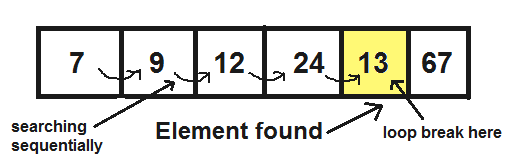# Linear Search( Java Implementation)

Linear search is a very simple search algorithm. In this type of search, a sequential search is made over all items one by one. Every item is checked and if a match is found then that particular item is returned, otherwise the search continues till the end of the data collection.For example, consider an array of integers of size N. You should find and print the position of all the elements with value x. Here, the linear search is based on the idea of matching each element from the beginning of the list to the end of the list with the integer x, and then printing the position of the element if the condition is True’.

#### Algorithm:

• Step 1: Traverse the array
• Step 2: Match the key element with array element
• Step 3: If key element is found, return the index position of the array element

Let’s see an example of linear search in java where we are going to search an element sequentially from an array.

//Java code for linearly search an item in an arr[].
//If item is present then it will return its index and location
//otherwise return -1
public class Search {

//this function returns index of element itemToSearch in arr[]
public int linearSearch(int arr[], int n, int item)
{
for (int i = 0; i <= n; i++)
{
//return the index of the element if it is found
if (item == arr[i])
return i;
}
return -1;
}

public static void main(String[] strr) {
int noOfItem, itemToSearch;
int arr[] = {7, 9, 12, 24, 13, 67};
noOfItem = arr.length - 1;
itemToSearch = 13;
int index = new Search().linearSearch(arr, noOfItem, itemToSearch);
if (index==-1)
System.out.println("item does not exist");
else
System.out.println("Item"+" " +itemToSearch+" " + "found at index :" + index + " and " +"position :" + (index+1));
}

}`

#### Output:

Item 13 found at index :4 and position :5

#### Time Complexity:

The time complexity of the linear search is O(N) because each element in an array is compared only once.

Linear search is rarely used practically because other search algorithms such as the binary search algorithm and hash tables allow significantly faster searching comparison to Linear search.